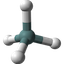Introduction to Molecules

Q

Where to find FAQ (Frequently Asked Questions) in understanding what is molecule and its basic properties?

✍: FYIcenter.com

AHere is a list of tutorials to answer many frequently asked questions compiled by FYIcenter.com team in understanding what is molecule.

What Is Molecule

What Is Molecular Formula

What Is Atomic Bond

What Is Molecule Structure

Skeletal Formula Notations

What Is Ion

What Are Valence Electrons

What Is Formal Charge